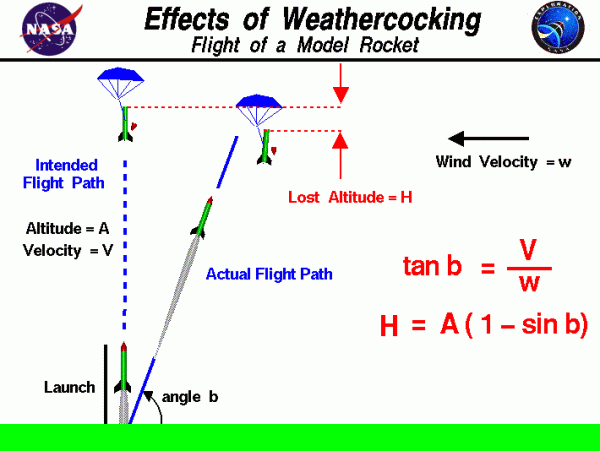# Effects of Weathercocking## Weathercocking

Following the liftoff of a model rocket, it often turns into the wind. This maneuver is called weathercocking and it is caused by aerodynamic forces on the rocket. Wind striking the side of the rocket generates a side force which acts through the center of pressure. For stability reasons, the center of pressure is located below the center of gravity of the rocket. The effect of the side force is to rotate the rocket about the center of gravity until the nose is inclined at the angle b to the horizontal. Angle b is the effective flow direction. If the wind velocity is w and the flight velocity is V, then:

$$\LARGE \tan b=\frac{V}{w}$$

where “tan” is the trigonometric tangent function.

## Rotation of Rocket

The rotation of the rocket produces a new flight path into the wind, as shown at the left of the figure. When the new flight path is aligned with the effective flow direction, there is no longer any lift force, and the rocket will continue to fly in the new flight direction. The flight path is inclined to the horizontal at angle b. The chief effect of weathercocking is that the maximum altitude of the flight is reduced. We can estimate the amount of lost altitude H by using some trigonometry. If the maximum vertical altitude is denoted by A, the lost altitude is given by:

$$\LARGE H=A(1-\sin b)$$

where “sin” is the trigonometric sine function. As a check, if the wind velocity is zero, the angle b is 90 degrees, and the lost altitude is zero.

Provide feedback